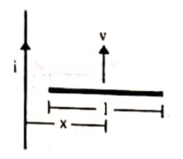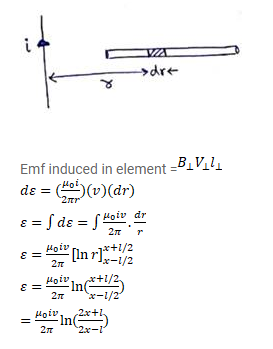# Figure shows a straight, long wire carrying a current i

Question:

Figure shows a straight, long wire carrying a current $i$ and a rod of length I coplanar with the wire and perpendicular to it. The rod moves with a constant velocity $v$ in a direction parallel to the wire. The distance of the wire from the center of the rod Find the motional emf induced in the rod.Solution: